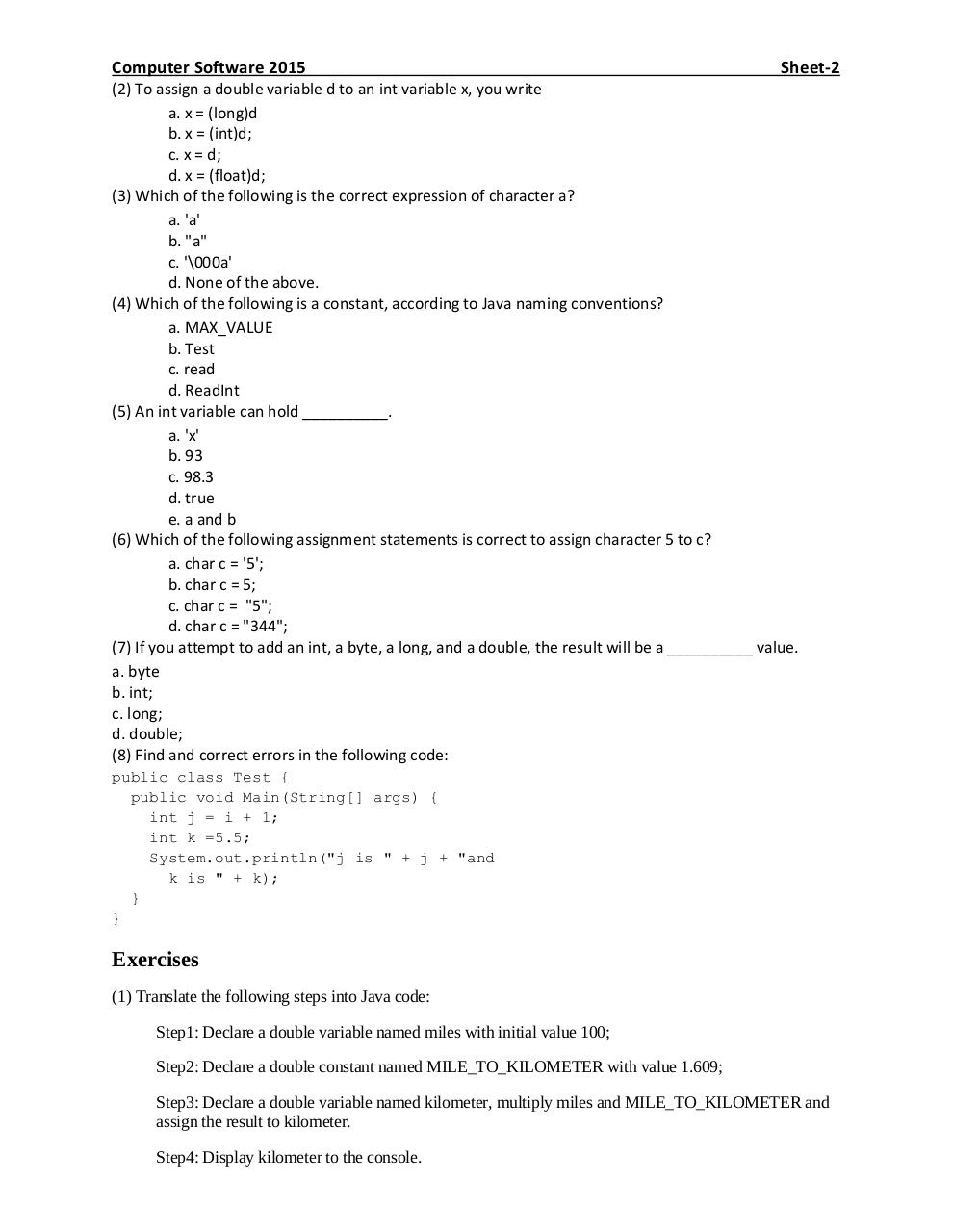# Sheet 2 2015.pdfPage 1 2 3 4 5 6 7

#### Text preview

Computer Software 2015

Sheet-2

(2) To assign a double variable d to an int variable x, you write
a. x = (long)d
b. x = (int)d;
c. x = d;
d. x = (float)d;
(3) Which of the following is the correct expression of character a?
a. 'a'
b. &quot;a&quot;
c. '\000a'
d. None of the above.
(4) Which of the following is a constant, according to Java naming conventions?
a. MAX_VALUE
b. Test
(5) An int variable can hold __________.
a. 'x'
b. 93
c. 98.3
d. true
e. a and b
(6) Which of the following assignment statements is correct to assign character 5 to c?
a. char c = '5';
b. char c = 5;
c. char c = &quot;5&quot;;
d. char c = &quot;344&quot;;
(7) If you attempt to add an int, a byte, a long, and a double, the result will be a __________ value.
a. byte
b. int;
c. long;
d. double;
(8) Find and correct errors in the following code:
public class Test {
public void Main(String[] args) {
int j = i + 1;
int k =5.5;
System.out.println(&quot;j is &quot; + j + &quot;and
k is &quot; + k);
}
}

Exercises
(1) Translate the following steps into Java code:
Step1: Declare a double variable named miles with initial value 100;
Step2: Declare a double constant named MILE_TO_KILOMETER with value 1.609;
Step3: Declare a double variable named kilometer, multiply miles and MILE_TO_KILOMETER and
assign the result to kilometer.
Step4: Display kilometer to the console.